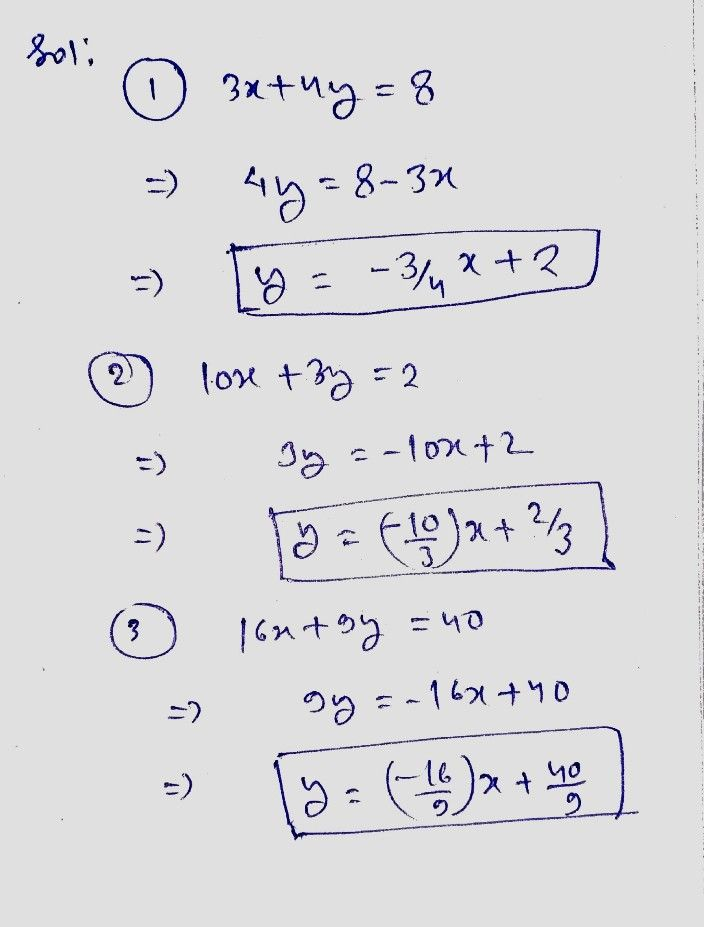Symbol
Problem$A$ Write the linear equation $ax+by=c$ in the form $y=mx+b$ $1.3x+4y=8$ $2$ $10x+3y=2$ $3.$ $16x+9y=40$
7th-9th grade
Other
Search count: 106
SolutionQanda teacher - Priya